## RS Aggarwal Class 10 Solutions Chapter 16 Co-ordinate Geometry Ex 16B

These Solutions are part of RS Aggarwal Solutions Class 10. Here we have given RS Aggarwal Solutions Class 10 Chapter 16 Co-ordinate Geometry Ex 16B.

Other Exercises

Question 1.
Solution:
(i) Let P (x, y) be the required point which divides the line joining the points A (-1, 7) and B (4, -3) in the ratio 2 : 3.Question 2.
Solution:
Let (7, -2) and B (1, -5) be the given points and P (x, y) and Q (x’, y’) are the points of trisection.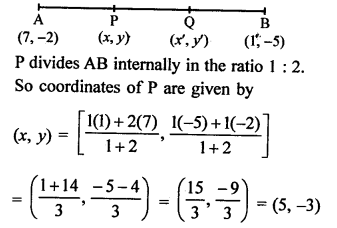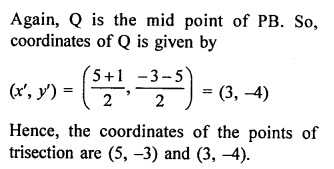Question 3.
Solution:
Let coordinates of P be (x, y) which divides the line segment A (-2, -2) and B (2, -4) in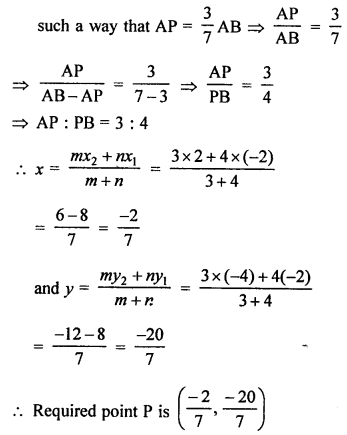Question 4.
Solution:
Let the coordinates of A be (x, y) which lies on line joining P (6, -6) and Q (-4, -1)Question 5.
Solution:
Points P, Q, R and S divides a line segment joining the points A (1, 2) and B (6, 7) in 5 equal parts.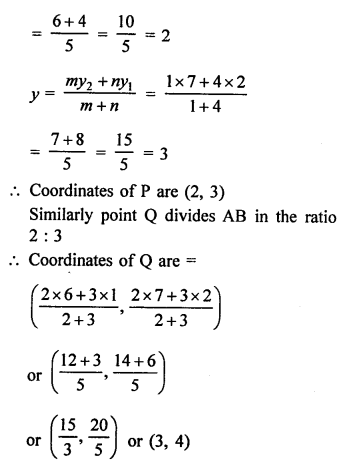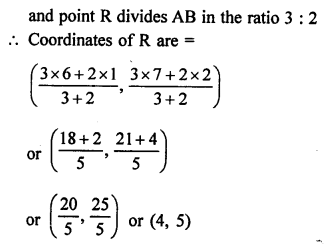Question 6.
Solution:
Points P, Q and R in order divide a line segment joining the points A (1, 6) and B (5, -2) in 4 equal parts.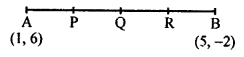P divides AB in the ratio of 1 : 3 Let coordinates of P be (x, y), thenQuestion 7.
Solution:
The line segment joining the point A (3, -4) and B (1, 2) is trisected by the points P (p, -2) and Q($$\frac { 1 }{ 2 }$$, q).Question 8.
Solution:
Mid point of the line segment joining the points A (3, 0) and B (-5, 4)Question 9.
Solution:
(2, p) is the mid point of the line segment joining the points A (6, -5), B (-2, 11)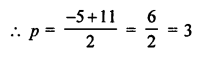Question 10.
Solution:
Mid point of the line segment joining the points A (2a, 4) and B (-2, 3b) is C (1, 2a + 1)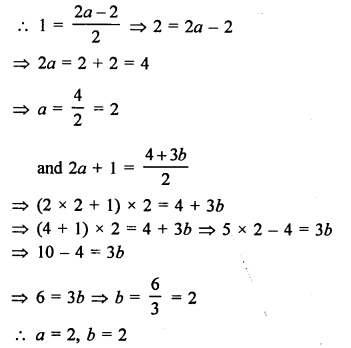Question 11.
Solution:
The line segment joining the points A (-2, 9) and B (6, 3) is a diameter of a circle with centre C.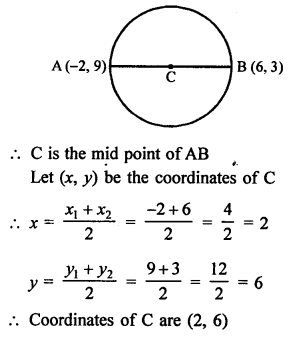Question 12.
Solution:
AB is diameter of a circle with centre C.
Coordinates of C (2, -3) and of B (1, 4)Question 13.
Solution:
Let P (2, 5) divides the line segment joining the points A (8,2) and B (-6, 9) in the ratio m : n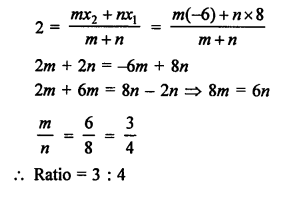Question 14.
Solution: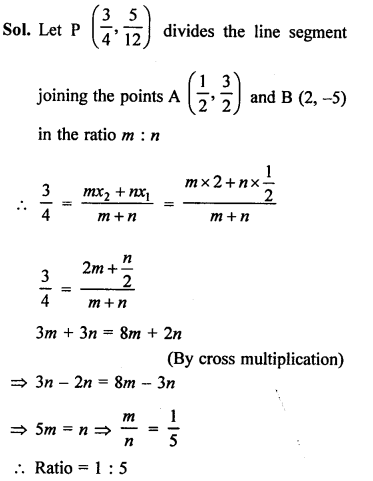Question 15.
Solution:
Let P (m, 6) divides the join of A (-4, 3) and B (2, 8) in the ratio k : 1
Then coordinates of P will be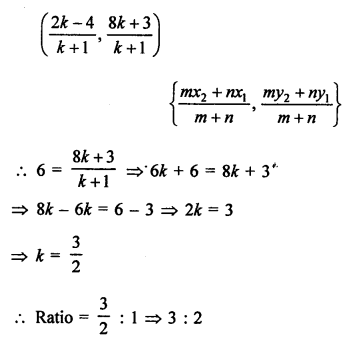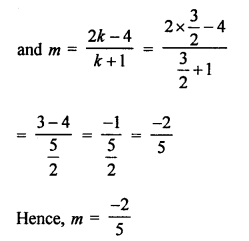Question 16.
Solution:
Let point P (-3, k) divides the join of A (-5, -4) and B (-2, 3) in the ratio m : n, then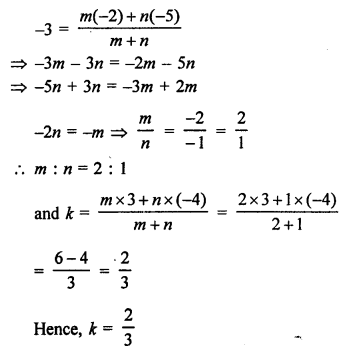Question 17.
Solution:
Let point P on the x-axis divides the line segment joining the points A (2, -3) and B (5, 6) the ratio m : n
Let P is the point on x-axis whose coordinates are (x, 0)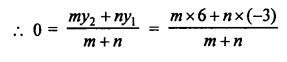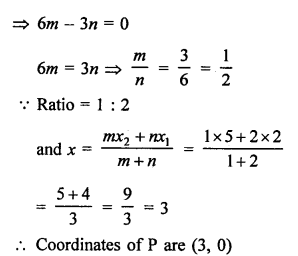Question 18.
Solution:
Let a point P on y-axis divides the line segment joining the points A (-2, -3) and B (3, 7) in the ratio m : n
Let the coordinates of P be (0, y)Question 19.
Solution:
Let a point P (x, y) on the given line x – y – 2 = 0 divides the line segment joining the points A (3, -1) and B (8, 9) in the ratio m : n, thenQuestion 20.
Solution:
Vertices of ∆ABC are A (0, -1), B (2, 1) and C (0, 3)
Let AD, BE and CF are the medians of sides BC, CA and AB respectively, then
Coordinates of D will be =Question 21.
Solution:
Centroid of ∆ABC where coordinates of A are (-1, 0), of B are (5, -2) and of C are (8, 2)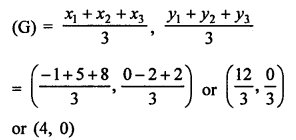Question 22.
Solution:
G (-2, 1) is the centroid of ∆ABC whose vertex A is (1, -6) and B is (-5, 2)
Let the vertex C be (x, y), thenQuestion 23.
Solution:
O (0, 0) is the centroid of ∆ABC in which B is (-3, 1), C is (0, -2)
Let A be (x, y), then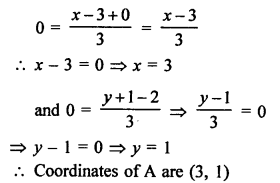Question 24.
Solution:
Points are A (3, 1), B (0, -2), C (1, 1) and D (4, 4)Question 25.
Solution:
Points P (a, -11), Q (5, b), R (2, 15) and S (1, 1) are the vertices of a parallelogram PQRS.
Diagonals of a parallelogram bisect each other.
O is mid point of PR and QS.Question 26.
Solution:
Three vertices of a parallelogram ABCD are A (1, -2), B (3, 6), C (5, 10).
Let fourth vertices D be (x, y)Question 27.
Solution:
Let a point P (0, y) on 7-axis, divides the line segment joining the points (-4, 7) and (3, -7) in the ratio m : n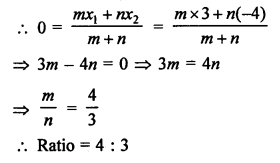Question 28.
Solution:Question 29.
Solution:
Let a point P (x, 0) divides the line segment joining the points A (3, -3) and B (-2, 2) in the ratio m : n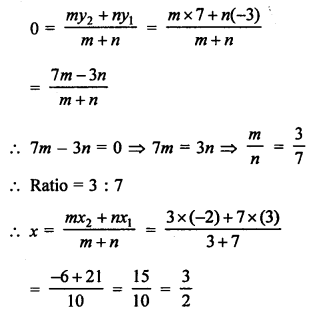Question 30.
Solution:
Base QR of an equilateral APQR lies on x- axis is O (0, 0) is mid point PQR and coordinate of Q are (-4, 0).
Coordinate of R will be (4, 0)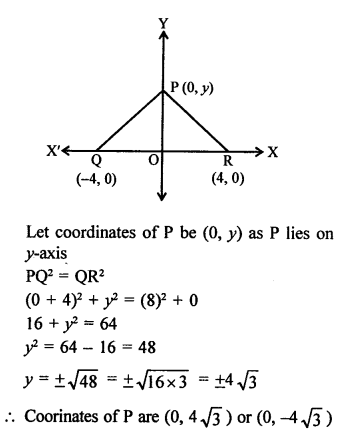Question 31.
Solution:
Base BC of an equilateral triangle ABC lies on y-axis in such a way that origin O (0, 0) lies is the middle of BC and coordinates of C are (0, -3).
Coordinates of B will be (0, 3)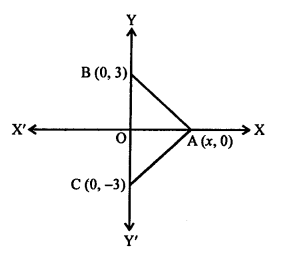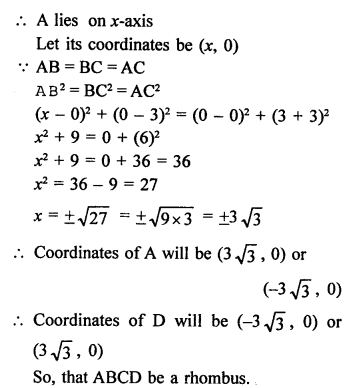Question 32.
Solution:
Let the points P (-1, y) lying on the line segment joining points A (-3, 10) and B (6, -8) divides it in the ratio m : n.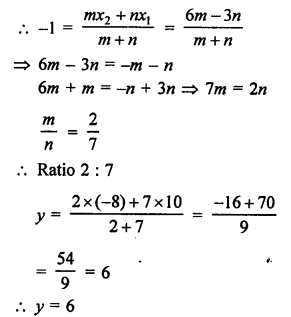Question 33.
Solution:
In rectangle ABCD, A (-1, -1), B (-1, 4), C (5, 4), D (5, -1)
P, Q, R and S are the mid points of AB, BC, CD and DA respectively.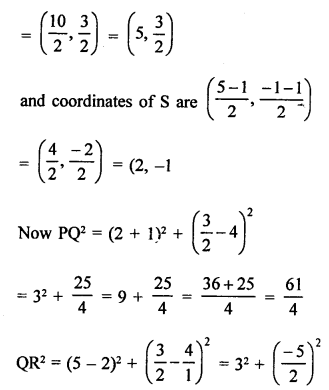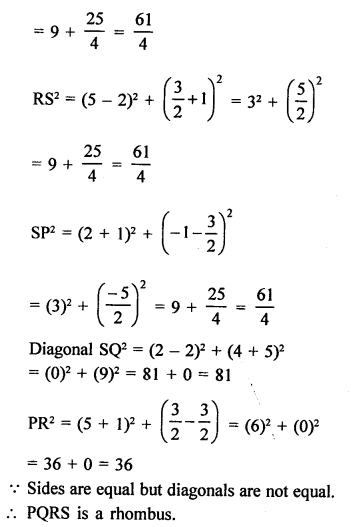Question 34.
Solution:
P is mid point of line segment joining the points A (-10, 4) and B (-2, 0)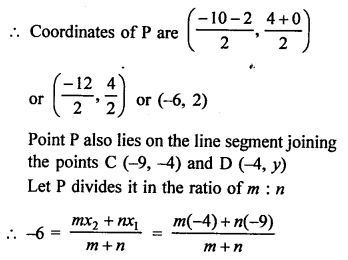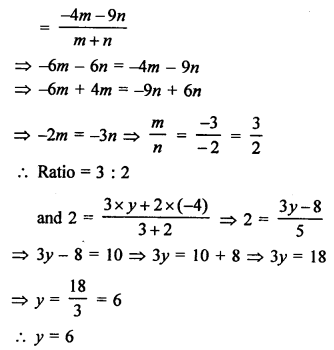Question 35.
Solution:
Let coordinates of P and Q be (0, y) and (x, 0) respectively.
Let M (2, -5) be the mid-point of PQ.
By midpoint formula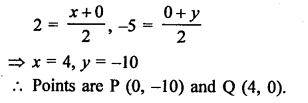Question 36.
Solution:Question 37.
Solution: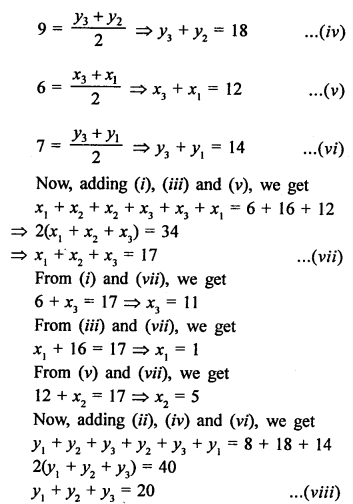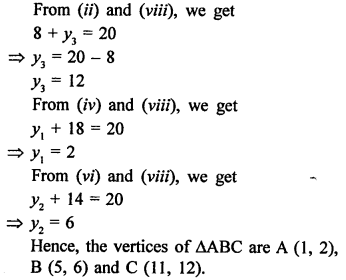Question 38.
Solution:
Let the other two vertices be (h, k) and (m, n).
Hence, the vertices in order are (3, 2), (-1, 0), (h, k) and (m, n).
It is to be kept in mind that the diagonals of a parallelogram bisect each other.
Hence, the point of intersection (2, -5) is the midpoint of the diagonal whose ends are (3, 2) and (h, k). ThenHope given RS Aggarwal Solutions Class 10 Chapter 16 Co-ordinate Geometry Ex 16B are helpful to complete your math homework.

If you have any doubts, please comment below. Learn Insta try to provide online math tutoring for you.